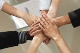# Getting value of field in template column onBlur

GridTop achievementsRank 2
David Penny asked on 26 Mar 2014, 05:01 PM
Hi,

I am trying to implement a row total function in Javascript for a RadGrid. Searching the help and forums I have managed to set up a function that is called by onBlur for each of my numeric columns, but cannot seem to get the value of the fields held in the template columns.

The definition of the columns (14 in total) is:

`<``telerik:GridTemplateColumn` `UniqueName``=``"colTime1"` `AllowFiltering``=``"False"` `HeaderText``=``"1"` `FooterStyle-Width``=``"50"` `HeaderStyle-Width``=``"50"` `ItemStyle-Width``=``"50"``>`
`                        ``<``ItemTemplate``>`
`                            ``<``asp:TextBox` `ID``=``"editTime1"` `runat``=``"server"` `Width``=``"40px"``></``asp:TextBox``>`
`                        ``</``ItemTemplate``>`
`                        ``<``FooterStyle` `Width``=``"50px"` `/>`
`                        ``<``HeaderStyle` `Width``=``"50px"` `/>`
`                        ``<``ItemStyle` `Width``=``"50px"` `/>`
`                    ``</``telerik:GridTemplateColumn``>`

And the javascript functin is:

`function valueChanged(totalTime, textbox, c, r) {`
`                   ``var grid = \$find("<%=RadGrid1.ClientID %>");`
`                   ``var MasterTable = grid.get_masterTableView();`
`                   ``var Rows = MasterTable.get_dataItems();`
`                   ``var valnow = textbox.value;`
`                   ``var total = valnow;`
`                   ``for (col = 1; col < 15; col++) {`
`                       ``if (col != c) {`
`                           ``var row = Rows[r - 1];`
`                           ``var columnName = "EditTime".concat(col);`
`                           ````var textTime = row.findControl(columnName); ```
`                           ``var colVal = textTime.get_value();`
`                           ``if (!isNan(colVal)) {`
`                               ``total += colVal;`
`                           ``}`
`                           ``else {`
`                               ``textTime.set_value("");`
`                           ``}`
`                       ``}`
`                   ``}`
`                   ``totalTime.set_value(total);`
`               ``}`

Everything is getting called OK but the lines:

`var row = Rows[r - 1];`
`var columnName = "EditTime".concat(col);`
`var textTime = row.findControl(columnName);`
`var colVal = textTime.get_value();`

Always returns null for textTime. The examples on the forum here seem to indicate the syntax is correct.

Am I implementing this wrong, or is there a better way of obtaining the values?

David Penny

## 6 Answers, 1 is accepted

0Princy
Top achievementsRank 1
answered on 27 Mar 2014, 09:26 AM
Hi David Penny,

Please take a look at the following code snippet to access the TextBox on onblur client event.

ASPX:
`<``telerik:GridTemplateColumn``>`
`    ``<``ItemTemplate``>`
`        ``<``asp:TextBox` `ID``=``"TextBox1"` `runat``=``"server"``></``asp:TextBox``>`
`    ``</``ItemTemplate``>`
`</``telerik:GridTemplateColumn``>`
`<``telerik:GridTemplateColumn``>`
`    ``<``ItemTemplate``>`
`        ``<``asp:TextBox` `ID``=``"TextBox2"` `runat``=``"server"``></``asp:TextBox``>`
`    ``</``ItemTemplate``>`
`</``telerik:GridTemplateColumn``>`

C#:
`protected` `void` `RadGrid1_ItemCreated(``object` `sender, GridItemEventArgs e)`
`{`
` ``if` `(e.Item ``is` `GridDataItem)`
`  ``{`
`   ``GridDataItem item = (GridDataItem)e.Item;`
`   ``//Accessing the TextBoxes of ItemTemplate`
`   ``TextBox txt1 = (TextBox)item.FindControl(``"TextBox1"``);`
`   ``TextBox txt2 = (TextBox)item.FindControl(``"TextBox2"``);`
`   ``txt1.Attributes.Add(``"onblur"``, ``"return Onblur('"` `+ txt1.ClientID + ``"','"` `+ txt2.ClientID + ``"')"``);`
`  ``}`
`}`

JS:
`<script type=``"text/javascript"``>`
`    ``function` `Onblur(txt1, txt2) {       `
`        ``var` `text1 = document.getElementById(txt1); ``// Access 1st textbox`
`        ``var` `text2 = document.getElementById(txt2); ``//Access 2nd textbox      `
`        ``text2.value = text1.value;``//set the textbox value`
`    ``}`
`</script>`

Thanks,
Princy
0David Penny
Top achievementsRank 2
answered on 27 Mar 2014, 10:04 AM
Hi Princy,

Thanks for a quick response, but that side of the code is already working. I can pass the control ID for the column I need to get a value to. What I am then trying to do is total all the OTHER column values (there are 14 daily columns).

Looking at the examples here it looks as though the following code would read the values from the row, where I pass the row ID to the javascript function.
`function valueChanged(totalTime, rowIndex) {`
`    ``var grid = \$find("<%=RadGrid1.ClientID %>");`
`    ``var MasterTable = grid.get_masterTableView();`
`    ``var Rows = MasterTable.get_dataItems();`
`    ``var total = 0;`
`    ``for (col = 1; col < 15; col++) {`
`            ``var row = Rows[rowIndex - 1];`
`            ``var columnName = "EditTime".concat(col);`
`            ``var textTime = row.findControl(columnName);`
`            ``var colVal = textTime.get_value();`
`            ``if (!isNan(colVal)) {`
`                ``total += colVal;`
`            ``}`
`        ``}`
`    ``}`
`    ``totalTime.set_value(total);`
`}`

The particular line of code that is failing me is:

var textTime = row.findControl(columnName);

Even if I hard code this as var textTime = row.findControl("EditTime1"); for example it always returns null in textTime.

I'm almost there but I need to be able to read the textboxes inside the template columns to perform the totaling.

David Penny

0Princy
Top achievementsRank 1
answered on 28 Mar 2014, 05:06 AM
Hi David Penny,

Please do the following modifications in your code snippet which works fine at my end.

JavaScript:
`...`
`var` `textTime = findElement(``"EditTime1"``); ``// accessing asp textbox`
`var` `colval=textTime.value; ``// value of the textbox`
`...`

Thanks,
Princy.
0David Penny
Top achievementsRank 2
answered on 28 Mar 2014, 03:25 PM
Hi Princy,

Should that be row.findElement()?

findElement() alone throws a null exception. And doesn't it need to know which row it's working on?

David Penny
0
AcceptedPrincy
Top achievementsRank 1
answered on 31 Mar 2014, 03:04 AM
Hi David Penny,

I apologize for giving you the wrong code. Please have a look into the sample code snippet which works fine at my end. Please let me know if you have any concern.

JavaScript:
`<script type=``"text/javascript"``>`
`    ``function` `OnValueChanged(sender, args) {`
`        ``var` `grid = \$find(``"<%=RadGrid1.ClientID %>"``);`
`        ``var` `total = 0;`
`        ``if` `(grid) {`
`            ``var` `MasterTable = grid.get_masterTableView();`
`            ``var` `Rows = MasterTable.get_dataItems();`
`            ``for` `(``var` `i = 0; i < Rows.length; i++) {`
`                ``//your code for adding the data`
`                ``var` `row = Rows[i];``//accessing each row of the grid`
`                ``var` `textTime = row.findElement(``"TextBox1"``); ``// accessing asp textbox`
`                ``var` `colval = parseInt(textTime.value); ``// value of the textbox`
`                ``total += colval;`
`            ``}`
`        ``}`
`    ``}`
`</script>`

Thanks,
Princy.
0David Penny
Top achievementsRank 2
answered on 31 Mar 2014, 09:01 AM
Thanks Princy - working great now. Marked as Answered.
Tags
GridDavid Penny
Top achievementsRank 2Rank 1Rank 2